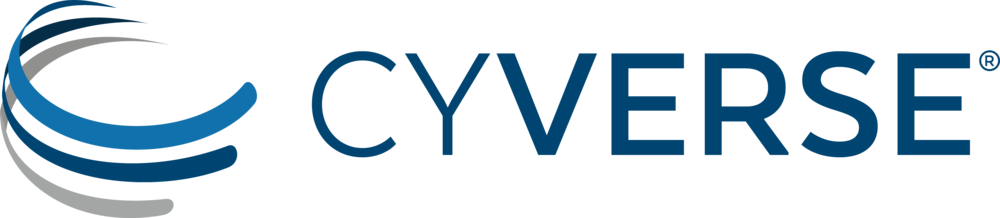# Introduction to R & RStudio¶

## Setup¶

2. Move to the Applications folder.
3. Open RStudio.

Go to Session -> Set Working Directory to set where you will pull data files from and/or save your code.

## Introduction¶

We will learn how to: - navigate & interact with R Studio

• UI of R Studio
• how to use “help”
• install packages
• data structures
• strings, factors, numbers, integers
• vectors & arrays
• matrices & lists
• explore data
• data manipulation
• data subsetting

R Studio makes using R programming language easier to interact with and to keep track of projects.

## Data Structures¶

### Types of Variables¶

Character - text that cannot have calculations done on them
e.g., “a”, “xyz”
Numeric - numerical values include decimals and can have calculations performed on them
e.g., 1, 1.5
Integer - whole numbers only, and can also have calculations performed on them
e.g., 2L (L stores it as an integer)

Logical - TRUE or FALSE

Exercise:

1. What does the following return? What does it mean?
str(10)
str("10")

Try calculations on the following.
1. What works and what doesn’t? Why or why not?
10*2
"10"*2

Errors v. Warnings:
Errors are given when R cannot perform the calculation Warnings mean that the function has run but perhaps with some issues.

### Storing Variables¶

We can assign any of the types of data above in a “place holder”. Variables are assignee using “<-“.

For example, we can store the number 10 in a letter to use later

a <- 10


NOTE Do not create variables that are already functions or arguments (e.g., c, T, F). NOTE Do not overwrite variables.

Exercise:

1. What does a*2 give you?

### Vectors¶

Vectors are 1-D object that contain “like” data types. You can create a string of variables and add to a vector using c(), which is short for concatenate.

Exercise:

1. What are the outputs of the code below?
2. Create your own vector using the vector() function.
x <- c(1, 2, 3, 4, 5)
y <- 1:5
z <- seq(1, 5, 1)

1. Are x, y, and z all the same structure? If not, how would you make them all the same?

Adding to vectors: the concatenate function: c()

d <- 1
d <- c(d, 2)

Try adding two to every numer in the vector “x”.
1. How do you add two to every number in x?

What happens what you add a character to a vector?

ATOMIC VECTORS are vectors which cannot be simplified anymore, and therefore “$” cannot be used on them. Yes, this error happens a lot. Yes, it is frustrating. Good luck. ### Matrices & Dataframes¶ A matrix and a dataframe are both 2-D objects that are made up of vectors. Creating a dataframe using data.frame() Exercise: 1. Play with the different types of data in the data.frame(). What happens? You can combine dataframes: hello <- data.frame (1:26, letters, words = c("hey", "you")) hi <- data.frame(1:26, letters, c("hey", "you")) howdy <- data.frame(hello, hi)  How do you name the column with the numbers 1-26? What are the column headers? What happends when you do the following? Adding columns and rows using cbind() and rbind() cbind(hello, "goodbye")  We can call columns using$ in the form of data.frame$column or call them using the modifier data.frame[row#, column#] Calling columns: hello[,2] #[] are like an index hello$letters


Subsetting:

Useful Functions to explore data types

View()  #can also double click on dataframe inside the R environment tab
str()
summary()
class()
typeof()
length()
attributes() #can also click on dataframe inside the R environment tab
dim()
tail()


Exercise

1. What is the output?
hello[,-2]


Likewise, columns and rows can be removed using “-” as a modifier

You can save a dataframe using write.table() and write.csv().

NOTE do not overwrite your dataset!!

If you rerun a script, you may overwrite your results or new data. Put a “#” after use!

### The R Environment¶

You can view your environment either by looking at the upper left tab or by typing the following:

ls() #see variables in your environment


You can remove objects using the rm() function.

Exercise:

1. How would you remove “a” from the environment? How would you check?

## Exploring Data¶

### Data Manipulation¶

Create the following dataframe:

cats <- data.frame(coat = c("calico", "black", "tabby"),
weight = c(2.1, 5.0,3.2),
likes_string = c(1, 0, 1))
class(cats)


cats$weight + 2 cats$coat + cats$coat  What are the outputs? We can use the function “paste” to make more complex strings: paste("My cat is", cats$coat)


What is the output?

### Subsetting Data¶

Exercise:

1. What is the function for subsetting data?
2. What are the outputs?
x <- c(a=5.4, b=6.2, c=7.1, d=4.8, e=7.5) # we can name a vector 'on the fly'
#x is a vector
x[c(a,c),]
x[names(x) == "a"]
x[names(x) == "a" | "c"]
x[names(x) != "a"]


## Terminal¶

Can run terminal in RStudio. This is useful if you want to run a program and still be able to use R, or if you need dependencies. Also, the terminal does not interact with the R environment.

Tools –> Terminal –> New Terminal